• 首先看看SVM的损失函数，梯度函数 损失函数最终形式： L = (1/N)∑iLi + λR(W) 其中： R(W) = ∑k∑s (Wk,s)2 Li = ∑j≠yi max(0, (xiW)j−(xyiW)j+Δ) 理解：yi表示提前知道第yi个分类是正确的。比yi大...
1.对于SVM这里总结下代码实现SVM的两种代码实现（for循环和矩阵操作）
首先看看SVM的损失函数，梯度函数
损失函数最终形式：
L = (1/N)∑iLi + λR(W)
其中：
R(W) = ∑k∑s (Wk,s)2
Li = ∑j≠yi max(0, (xiW)j−(xyiW)j+Δ)
理解：yi表示提前知道第yi个分类是正确的。比yi大的评分表示判断错的得分，因为如果正确，第yi个分类的得分应该是最高的。

梯度函数最终形式：
∇Wyi Li = - xiT(∑j≠yi 1(xiWj - xiWyi +1>0)) + 2λWyi    （j = y[i]）
∇Wj Li = xiT 1(xiWj - xiWyi +1>0) + 2λWj , (j≠yi)         (j != y[i])

其中，注意1(·)是示性函数，其取值规则为：1(表达式为真) =1；1(表达式为假) =0。
理解：这里分了两种情况，当计算dW矩阵的时候，计算每一张图的dW矩阵，第y[i]列的计算要注意（我觉得这里不好懂），计算其他列的计算比较好理解。
下面先看for循环的实现代码：

def svm_loss_naive(W, X, y, reg):

Inputs:
- W: A numpy array of shape (D, C) containing weights.
- X: A numpy array of shape (N, D) containing a minibatch of data.
- y: A numpy array of shape (N,) containing training labels; y[i] = c means
that X[i] has label c, where 0 <= c < C.
- reg: (float) regularization strength

Returns a tuple of:
- loss as single float
- gradient with respect to weights W; an array of same shape as W
"""
dW = np.zeros(W.shape) # initialize the gradient as zero

# compute the loss and the gradient
num_classes = W.shape
num_train = X.shape
loss = 0.0
for i in xrange(num_train):
scores = X[i].dot(W)
correct_class_score = scores[y[i]]
for j in xrange(num_classes):
if j == y[i]:
continue
margin = scores[j] - correct_class_score + 1 # note delta = 1
if margin > 0:#也就是只有1(xiWj - xiWyi +1>0)的时候才进行loss和gradient的计算
loss += margin
dW[:,y[i]]+= -X[i,:]
dW[:,j] += X[i,:]
#上面两行代码这样理解，每张图片输入，就是i循环一次，对应的j循环了num_class次，就是把dW矩阵的每一列都遍历到了，（也就是每张图对应一个dW，i循环完了，就是将num_train个dW矩阵叠加了一遍），这里dW[:,y[i]]+= -X[i,:]在每一次i循环都执行，就是第y[i]列是其他列的和，其他列只循环了一次。
if margin > 0:表示有错误的评分出现，这个时候才会产生损失值，所以这里才会有loss的计算

# Right now the loss is a sum over all training examples, but we want it
# to be an average instead so we divide by num_train.
loss /= num_train
dW = dW/num_train
# Add regularization to the loss.
loss += 0.5*reg * np.sum(W * W)
dW = dW+ reg*W
return loss, dW


下面看看矩阵操作的实现：

def svm_loss_vectorized(W, X, y, reg):

loss = 0.0
dW = np.zeros(W.shape) # initialize the gradient as zero
num_classes = W.shape
num_train = X.shape
#############################################################################
# TODO:                                                                     #
# Implement a vectorized version of the structured SVM loss, storing the    #
# result in loss.                                                           #
#############################################################################
scores = X.dot(W)
score_correct = scores[np.arange(num_train),y]#根据已知的y，找出本应该得分最高的分类得分，存放入一个矩阵，这个矩阵大小【1*N】

score_correct = np.reshape(score_correct,(num_train,1))#必须这样才能把矩阵变为[N*1],不能用转置，python中转置操作对以为矩阵不起作用（有点坑）
margin_matrix = scores - score_correct +1#矩阵化实现(xiW)j−(xyiW)j+Δ

margin_matrix[np.arange(num_train),y] =0 #因为loss公式中指明j=y[i]的列不参与计算，因为这一列是本应该分类正确的，其他列都是和这一列作比较。

margin_matrix[margin_matrix<=0] =0

loss = np.sum(margin_matrix)#计算所有loss的和

loss /= num_train
loss = loss + 0.5*reg*np.sum(W*W)

#############################################################################
#                             END OF YOUR CODE                              #
#############################################################################

#############################################################################
# TODO:                                                                     #
# Implement a vectorized version of the gradient for the structured SVM     #
# loss, storing the result in dW.                                           #
#                                                                           #
# Hint: Instead of computing the gradient from scratch, it may be easier    #
# to reuse some of the intermediate values that you used to compute the     #
# loss.                                                                     #
#############################################################################
margin_matrix[margin_matrix>0] =1#这一步是对用梯度公式中 1(xiWj - xiWyi +1>0))  这一项

row_sum = np.sum(margin_matrix, axis=1)#将所有非正确分类，且这个非正确分类的评分要大于正确的评分，做个求和
margin_matrix[np.arange(num_train), y] = -row_sum
#上面两句代码对应for循环中的dW[:,y[i]]+= -X[i,:]这一句
dW = np.dot(X.T, margin_matrix) / num_train + reg * W
#然后根据矩阵的对应关系进行计算，就是维度关系
return loss, dW


后面会根据梯度下降发来跟新W
其实代码就一句话

self.W += -learning_rate * grad

只是这里的grad一般使用很小的batch来计算的，为了加快运算速度而已。
展开全文svm python
• 首先来看看softmax的损失函数和梯度函数公式 结合惩罚项，给出总的损失函数： L = -(1/N)∑i∑j1(k=yi)log(exp(fk)/∑j exp(fj)) + λR(W) 下面有几个准备函数也要理解： Li = -log(exp(fyi)/∑j exp(fj)) ：...
softmax也是一个用于多分类的线性分类器。
首先来看看softmax的损失函数和梯度函数公式
结合惩罚项，给出总的损失函数：
L = -(1/N)∑i∑j1(k=yi)log(exp(fk)/∑j exp(fj)) + λR(W)
下面有几个准备函数也要理解：
Li = -log(exp(fyi)/∑j exp(fj)) ：这个就是最基本的softmax函数，也就是本应该正确的分类得分在所有的类得分的比例，也可以说概率。
在实际编程计算softmax函数时，可能会遇到数值稳定性（Numeric stability）问题（因为在计算过程中，exp(fyi) 和 ∑j exp(fj) 的值可能会变得非常大，大值数相除容易导致数值不稳定），为了避免出现这样的问题，我们可以进行如下处理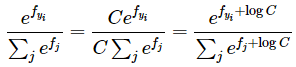其中，C的取值通常为：logC = -maxj fj，即-logC取f每一行中的最大值。
现在，结合惩罚项，给出总的损失函数：
L = -(1/N)∑i∑j1(k=yi)log(exp(fk)/∑j exp(fj)) + λR(W)
理解：其实就是图片矩阵得X，每一行中本该得分正确的得分的值除以那一行所有的类的得分和，得到一个概率，在把每一行得到的概率加起来，置于公式里面的exp，log加上取就好，logC的引入也就解释，就是为了数值稳定性。

然后是梯度求解：
这里粘贴一个求解梯度的推导过程：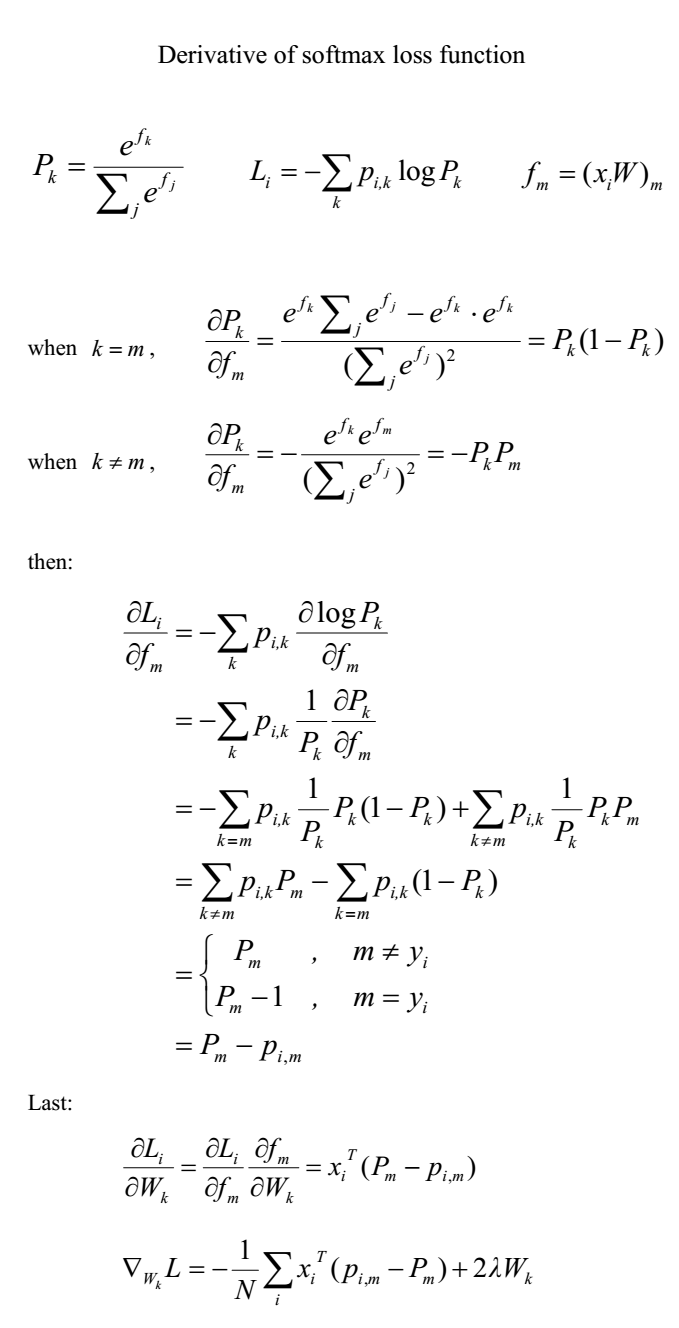这里面的pi,m,当样本分类正确时候，取1，其他元素取0，pm为p[i,m],表示第i张图片在第m个分类上的概率。确实这里（pi,m-Pm）要明白怎么代码实现。
下面看看For循环代码

def softmax_loss_naive(W, X, y, reg):

Inputs:
- W: A numpy array of shape (D, C) containing weights.
- X: A numpy array of shape (N, D) containing a minibatch of data.
- y: A numpy array of shape (N,) containing training labels; y[i] = c means
that X[i] has label c, where 0 <= c < C.
- reg: (float) regularization strength

Returns a tuple of:
- loss as single float
- gradient with respect to weights W; an array of same shape as W
"""
# Initialize the loss and gradient to zero.
loss = 0.0
dW = np.zeros_like(W)

#############################################################################
# TODO: Compute the softmax loss and its gradient using explicit loops.     #
# Store the loss in loss and the gradient in dW. If you are not careful     #
# here, it is easy to run into numeric instability. Don't forget the        #
# regularization!                                                           #
#############################################################################
num_train = X.shape
num_class = W.shape
print (num_train,':',num_class)
scores = X.dot(W)
scores_max = np.reshape(np.max(scores,axis =1),(num_train,1))
prob = np.exp(scores - scores_max)/np.sum(np.exp( scores - scores_max ),axis =1,keepdims =True)#这里的keepdims必须加上，否则不同维度的矩阵不能进行操作。
#N*C
correct_prob = np.zeros_like(prob)

correct_prob[np.arange(num_train),y] =1

for i in range(num_train):
for j in range(num_class):
loss += -( correct_prob[i,j]* np.log(prob[i,j]) )
dW[:,j] += (prob[i,j] - correct_prob[i,j])*X[i,:]
# if j==y[i]:
#     loss += (-np.log(prob[i,j]))
#     dW[:,j] -=(1-prob[i,j])*X[i,:]
# else:
#     dW[:,j] -= (0-prob[i,j]) * X[i]
#上面求梯度的两行代码，j就相当于公式中的m,遍历所有的分类，得到一张图对应的一个[N*C]权重矩阵dW，然后讲每个i对应的dW相加。
loss = loss/num_train
loss = loss + 0.5*reg*np.sum(W*W)
dW = dW/num_train
dW += reg*W
return loss, dW


下面看看矩阵操作实现：

def softmax_loss_vectorized(W, X, y, reg):

# Initialize the loss and gradient to zero.
loss = 0.0
dW = np.zeros_like(W)

#############################################################################
# TODO: Compute the softmax loss and its gradient using no explicit loops.  #
# Store the loss in loss and the gradient in dW. If you are not careful     #
# here, it is easy to run into numeric instability. Don't forget the        #
# regularization!                                                           #
#############################################################################
num_train = X.shape
scores = X.dot(W)
scores_max= np.max(scores,axis =1)
scores_max = np.reshape(scores_max,(num_train,1))
scores_correct  = scores[np.arange(num_train), y]

scores_1 = scores -scores_max
scores_correct_1 =scores_correct -scores_max

prob = np.exp(scores_1) /np.sum(np.exp(scores_1),axis =1,keepdims= True)#caculatie the problity of each score N*C
keep_prob = np.zeros_like(prob)
keep_prob[np.arange(num_train),y] =1

#prob =np.log(np.exp(scores_correct_1)) / np.sum((np.log(np.exp(scores_1)
# loss = np.sum( np.log(np.exp(scores_correct_1)) / np.sum((np.log(np.exp(scores_1))),1) )
loss += np.sum(keep_prob*np.log(prob ))
loss =loss/num_train*(-1)
loss =loss + 0.5*reg*np.sum(W*W)
# print ('loss1:',loss)
dW += X.T.dot(keep_prob - prob) #这一句话就是最重要的一句话。也是softmax矩阵操作核心
dW = -dW/num_train+reg*W
# dW += -np.dot(X.T,keep_prob -prob)/num_train +reg*W

return loss, dW


有了loss和gradient，通过梯度下降法来优化权重矩阵W了。

self.W += -learning_rate*grad

也就是这句话。
好了，这两个算法吧还要慢慢在实际使用中慢慢加深了理解。
展开全文• System.out.println("请输入算数表达式："); str = input.nextLine(); newStr = str + "#"; E(); System.out.println("正确语句！"); } public static void E() { T(); X(); } public static ...
以Sample语言为例，对于给定的文法G[E]

E->TX
X->+TX | ε;
T->FY;
Y->*FY | ε;

F->(E) | i

采用递归下降语法分析法编写语法分析程序，该语法分析程序判断输入的字符串是否符合上述文法，并能够输出相应的结果（是语法成分或不是语法成分）。

具体实现方式如下（java实现）。

/**
*
* @author Sun
*
*/
public class GradientDecsent {

private static String str;
private static int index;
private static String newStr;

public static void main(String[] ars) {
@SuppressWarnings("resource")
Scanner input = new Scanner(System.in);
System.out.println("请输入算数表达式：");
str = input.nextLine();
newStr = str + "#";
E();
System.out.println("正确语句！");
}

public static void E() {
T();
X();
}

public static void X() {
if (newStr.charAt(index) == '+') {
index++;
T();
X();
}
}

public static void T() {
F();
Y();
}

public static void Y() {
if (newStr.charAt(index) == '*') {
index++;
F();
Y();
}
}

public static void F() {
if (Character.isDigit(newStr.charAt(index)) || Character.isLetter(newStr.charAt(index))) {
index++;
} else if (newStr.charAt(index) == '(') {
index++;
E();
if (newStr.charAt(index) == ')') {
index++;
} else {
System.out.println("分析失败!\n");
System.exit(0);
}
} else {
System.out.println("分析失败!\n");
System.exit(0);
}
}
}


展开全文编译原理
• BP神经网络网络梯度的推导，显示的表达式 其实就是复合函数求导， https://www.bilibili.com/video/av10590361/?p=14 最近看李宏毅老师推导的神经网络网络的推导，讲的很好，但是没有写出显示的表达式，我这里...
神经网络网络梯度的推导（BP），显示的表达式
其实就是复合函数求导，
https://www.bilibili.com/video/av10590361/?p=14
最近看李宏毅老师推导的神经网络网络的推导，讲的很好，但是没有写出显示的表达式，我这里推导了详细的表达式：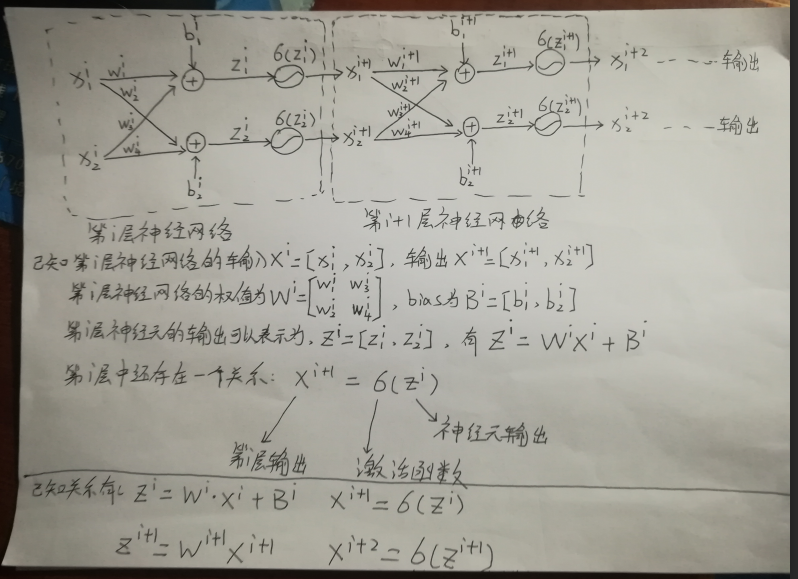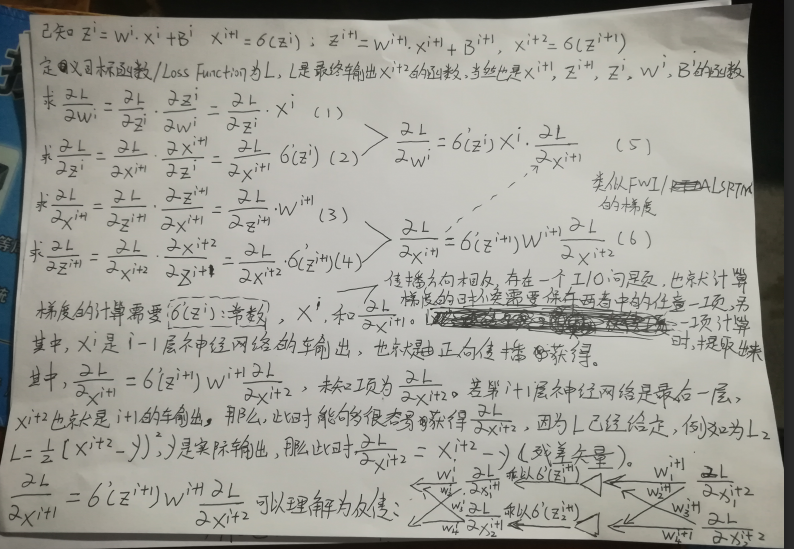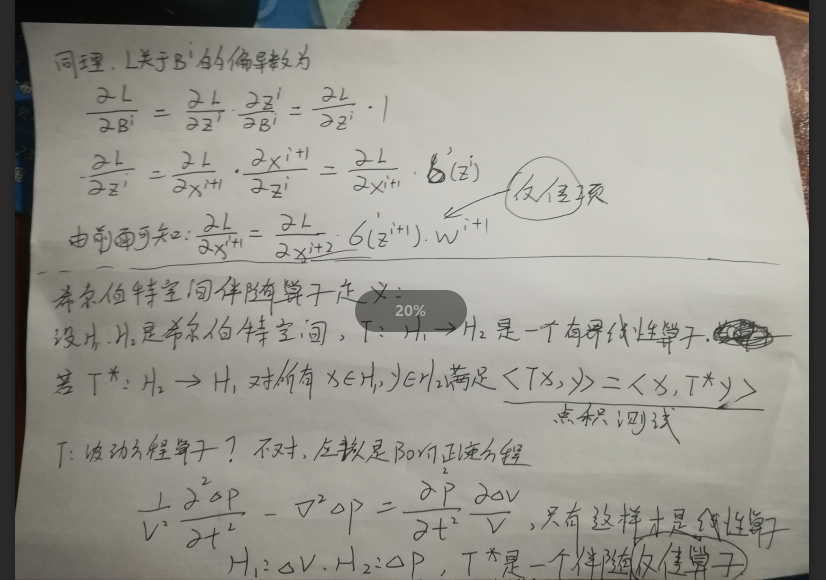展开全文机器学习 神经网络 链式法则
• 我们也花了很长时间来推导此公式，但是对于一些复杂的函数如非线性函数，求解梯度并不容易，在这种情况下，为了保证求解的梯度表达式正确，发现求解过程中的错误，需要进行梯度下降法的调试 定义\(\theta=(\theta_0...
• （代码差异包）是在计算机程序中进行梯度评估的工具。 它支持以下功能： 算法微分（AD）的前向模式 算法微分（AD）的反向模式 不同的磁带实现 AdjointMPI接口 外部功能 高阶导数 CoDiPack的设计原理是易于使用。 ...C++
• 1、首先根据loss函数对各个需要优化的参数（也就是权重，下同）求偏导，也就是求梯度表达式，最终得到各个参数的梯度表达式； 2、开始对模型进行训练时，首先应初始化参数，将初始化之后的参数以及第一个训练样本代...
• Template.h 是各种表达式模板的集合，包含必要的向量加减乘法操作符重载。 Matrix.h 是AX=b中,关键A数组生成的类，这里我用了数组压缩技术，即把带状数组A压缩，使程序执行更有效率。 cgexpr.cpp是Main函数，包括...
• 以开关动态系统的时间最优控制问题作为研究对象，给出了代价泛函的梯度表达式及证明过程，根据具有Armijo步长梯度下降算法得到数值解。通过仿真结果可以看出第一开关点为0.943 2 s，第二开关点为1.426 7 s，精度较高...
• plot function figure based on math equation https://www.transum.org/Maths/Activity/Graph/Desmos.asp
• 首先先明确一下梯度的概念，我们先从简单入手，假设有一个二元函数f(x,y)，这个函数的梯度表达式如下： 我们可以注意到，这个表达式相当于将关于x和y的一个向量映射为了x关于f(x,y)的偏导和y关于f(x,y)的偏导的一个...
•特征工程 模型参数 机器学习
• 原因一：Hessian矩阵难以求解。深度网络很难写出拟合函数的表达式，遑论直接得到其梯度表达式，更不要说得到基于梯度的Hessian矩阵了。
• 1 梯度下降法 函数在某一点的梯度是，在该方向单位步长上升最快的向量。梯度下降法是利用待优化变量，沿着负梯度方向不断迭代寻找最优值。 直观理解： ...梯度下降法证明：通过泰勒展开表达式证明沿...
• 一、结构 二、作用 1.在网络继续加深时，在训练集上的效果反而下降，而这...梯度表达式原本是连乘的形式，残差结构相当于将每层里的乘法变成了加法形式，考虑（1+0.01）*（1+0.02）*（1+0.01）...即使0.01部分...
• 文章目录提出问题暴力求解评价函数梯度曲线学习率 提出问题 已知如下图形在[0，10]区间内呈现高斯分布特征，在已知高斯公式表达式为： p(z)=12πσe(−(z−μ)22σ2) p(z) = \frac{1}{ \sqrt {2 \pi } \sigma } e^(-...
• 今天开始学习机器学习，遇到梯度问题，自己理解的如下； 梯度就是一个函数在某点各个方向的导数（偏导数）构成的向量，梯度的方向就是导数最大对应的那个方向；他的模为方向导数的最大...在三维情况，该表达式在直...
• 导数使用T.grad计算。...第3行输出是打印了经过优化器简化的符号梯度表达式，与第1个输出相比确实简单多了。 fill((x** TensorConstant{2}), TensorConstant{1.0})指创建一个x**2大小的矩阵，并填充1...
• 损失函数梯度 均方误差函数梯度 均方误差表达式梯度导数： 交叉熵函数梯度 Softmax 梯度 回顾 Softmax 函数的表达式： Softmax 梯度为： 交叉熵梯度 考虑交叉熵损失函数的表达式： 将log分解： 最终简化求...
• ## 梯度消失

千次阅读 2018-06-29 15:45:26
梯度消失是传统神经网络训练中非常致命的一个问题，其本质是由于链式法则的乘法特性导致的。比如之前神经网络中最流行的激活函数之一Sigmoid，其表达式如下：...
• 假设每一层神经元的表达式如下所示，激活函数为sigmoid函数 可以推导出： 是sigmoid函数的倒数图像为： 可以看到导函数的最大值为0.25，通常初始化的权值|w|<1，所以： 由于连乘，所以上式子 变得越小。...自然语言处理
• 表达式为： 若要对于w1求梯度，根据链式求导法则，得到的解为： 通常，若使用的激活函数为sigmoid函数，其导数： 这样可以看到，如果我们使用标准化初始w，那么各个层次的相乘都是0-1之间的小数，...
• 估计搞研究的人应该经常听见这个算法吧，用来求解表达式最大或者最小值的，属于无约束优化问题。 首先我们应该清楚，一个多元函数的梯度方向是该函数值增大最陡的方向。具体化到1元函数中时，梯度方向首先是沿着曲......